Anzeige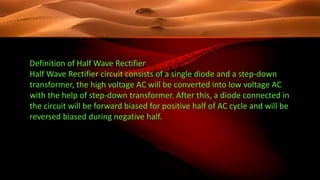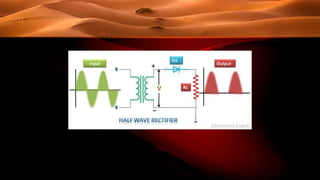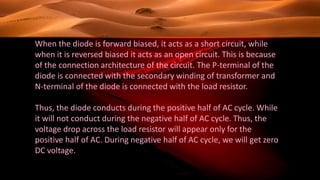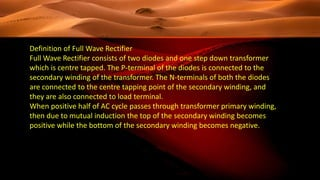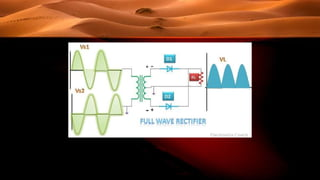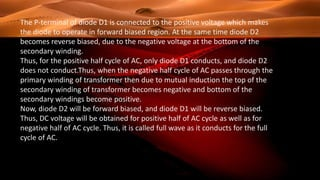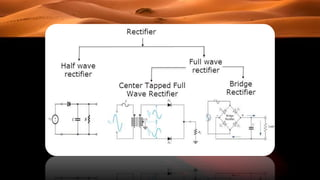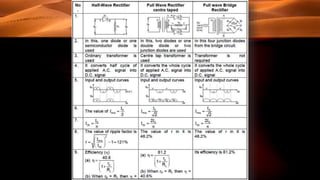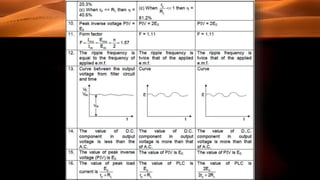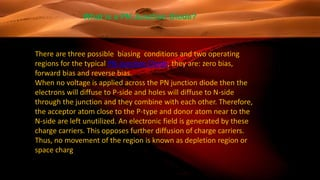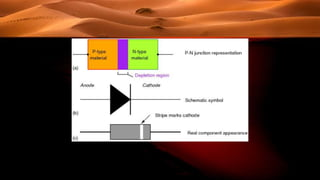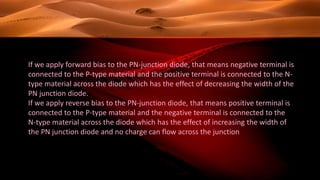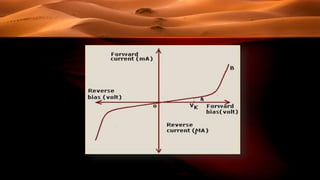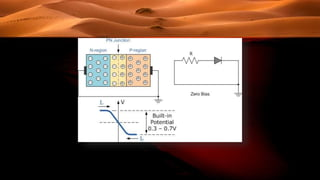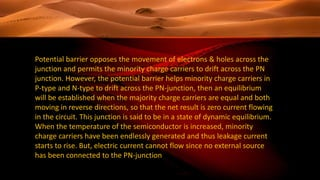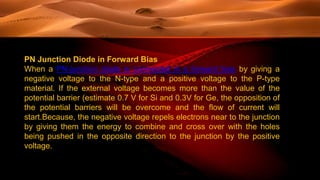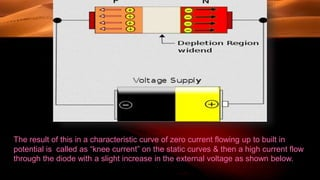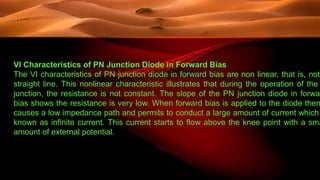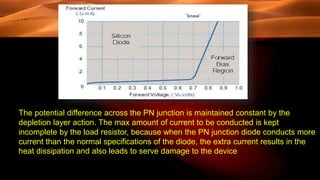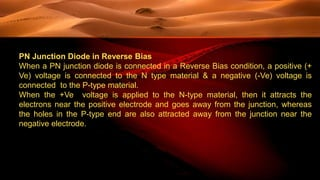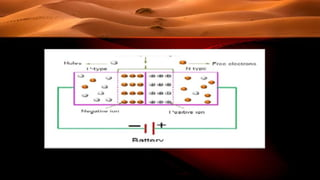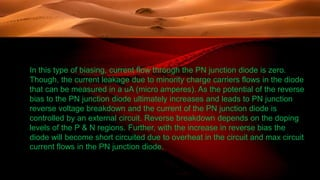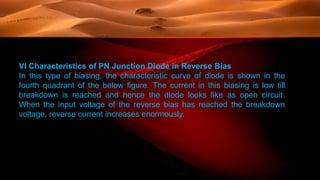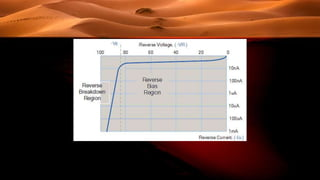1 von 26
Anzeige

### Difference between half wave and full wave rectifier

1. AL-AMIN
2. Definition of Half Wave Rectifier Half Wave Rectifier circuit consists of a single diode and a step-down transformer, the high voltage AC will be converted into low voltage AC with the help of step-down transformer. After this, a diode connected in the circuit will be forward biased for positive half of AC cycle and will be reversed biased during negative half.
3. When the diode is forward biased, it acts as a short circuit, while when it is reversed biased it acts as an open circuit. This is because of the connection architecture of the circuit. The P-terminal of the diode is connected with the secondary winding of transformer and N-terminal of the diode is connected with the load resistor. Thus, the diode conducts during the positive half of AC cycle. While it will not conduct during the negative half of AC cycle. Thus, the voltage drop across the load resistor will appear only for the positive half of AC. During negative half of AC cycle, we will get zero DC voltage.
4. Definition of Full Wave Rectifier Full Wave Rectifier consists of two diodes and one step down transformer which is centre tapped. The P-terminal of the diodes is connected to the secondary winding of the transformer. The N-terminals of both the diodes are connected to the centre tapping point of the secondary winding, and they are also connected to load terminal. When positive half of AC cycle passes through transformer primary winding, then due to mutual induction the top of the secondary winding becomes positive while the bottom of the secondary winding becomes negative.
5. The P-terminal of diode D1 is connected to the positive voltage which makes the diode to operate in forward biased region. At the same time diode D2 becomes reverse biased, due to the negative voltage at the bottom of the secondary winding. Thus, for the positive half cycle of AC, only diode D1 conducts, and diode D2 does not conduct.Thus, when the negative half cycle of AC passes through the primary winding of transformer then due to mutual induction the top of the secondary winding of transformer becomes negative and bottom of the secondary windings become positive. Now, diode D2 will be forward biased, and diode D1 will be reverse biased. Thus, DC voltage will be obtained for positive half of AC cycle as well as for negative half of AC cycle. Thus, it is called full wave as it conducts for the full cycle of AC.
6. What is a PN Junction Diode? There are three possible biasing conditions and two operating regions for the typical PN-Junction Diode, they are: zero bias, forward bias and reverse bias. When no voltage is applied across the PN junction diode then the electrons will diffuse to P-side and holes will diffuse to N-side through the junction and they combine with each other. Therefore, the acceptor atom close to the P-type and donor atom near to the N-side are left unutilized. An electronic field is generated by these charge carriers. This opposes further diffusion of charge carriers. Thus, no movement of the region is known as depletion region or space charg
7. If we apply forward bias to the PN-junction diode, that means negative terminal is connected to the P-type material and the positive terminal is connected to the N- type material across the diode which has the effect of decreasing the width of the PN junction diode. If we apply reverse bias to the PN-junction diode, that means positive terminal is connected to the P-type material and the negative terminal is connected to the N-type material across the diode which has the effect of increasing the width of the PN junction diode and no charge can flow across the junction
8. Zero Biased PN Junction Diode In the zero bias junction, potential provides higher potential energy to the holes on the P and N side terminals. When the terminals of the junction diode are shorted, few majority charge carriers in the P-side with plenty energy to overcome the potential barrier to travel across the depletion region. Therefore, with the help of majority charge carriers, the current starts to flow in the diode and it is denoted to as forward current. In the same way, minority charge carriers in the N-side move across the depletion region in reverse direction and it is referred to as reverse current.
9. Potential barrier opposes the movement of electrons & holes across the junction and permits the minority charge carriers to drift across the PN junction. However, the potential barrier helps minority charge carriers in P-type and N-type to drift across the PN-junction, then an equilibrium will be established when the majority charge carriers are equal and both moving in reverse directions, so that the net result is zero current flowing in the circuit. This junction is said to be in a state of dynamic equilibrium. When the temperature of the semiconductor is increased, minority charge carriers have been endlessly generated and thus leakage current starts to rise. But, electric current cannot flow since no external source has been connected to the PN-junction
10. PN Junction Diode in Forward Bias When a PN-junction diode is connected in a forward bias by giving a negative voltage to the N-type and a positive voltage to the P-type material. If the external voltage becomes more than the value of the potential barrier (estimate 0.7 V for Si and 0.3V for Ge, the opposition of the potential barriers will be overcome and the flow of current will start.Because, the negative voltage repels electrons near to the junction by giving them the energy to combine and cross over with the holes being pushed in the opposite direction to the junction by the positive voltage.
11. The result of this in a characteristic curve of zero current flowing up to built in potential is called as “knee current” on the static curves & then a high current flow through the diode with a slight increase in the external voltage as shown below.
12. VI Characteristics of PN Junction Diode in Forward Bias The VI characteristics of PN junction diode in forward bias are non linear, that is, not straight line. This nonlinear characteristic illustrates that during the operation of the junction, the resistance is not constant. The slope of the PN junction diode in forwar bias shows the resistance is very low. When forward bias is applied to the diode then causes a low impedance path and permits to conduct a large amount of current which known as infinite current. This current starts to flow above the knee point with a sma amount of external potential.
13. The potential difference across the PN junction is maintained constant by the depletion layer action. The max amount of current to be conducted is kept incomplete by the load resistor, because when the PN junction diode conducts more current than the normal specifications of the diode, the extra current results in the heat dissipation and also leads to serve damage to the device
14. PN Junction Diode in Reverse Bias When a PN junction diode is connected in a Reverse Bias condition, a positive (+ Ve) voltage is connected to the N type material & a negative (-Ve) voltage is connected to the P-type material. When the +Ve voltage is applied to the N-type material, then it attracts the electrons near the positive electrode and goes away from the junction, whereas the holes in the P-type end are also attracted away from the junction near the negative electrode.
15. In this type of biasing, current flow through the PN junction diode is zero. Though, the current leakage due to minority charge carriers flows in the diode that can be measured in a uA (micro amperes). As the potential of the reverse bias to the PN junction diode ultimately increases and leads to PN junction reverse voltage breakdown and the current of the PN junction diode is controlled by an external circuit. Reverse breakdown depends on the doping levels of the P & N regions. Further, with the increase in reverse bias the diode will become short circuited due to overheat in the circuit and max circuit current flows in the PN junction diode.
16. VI Characteristics of PN Junction Diode in Reverse Bias In this type of biasing, the characteristic curve of diode is shown in the fourth quadrant of the below figure. The current in this biasing is low till breakdown is reached and hence the diode looks like as open circuit. When the input voltage of the reverse bias has reached the breakdown voltage, reverse current increases enormously.
Anzeige